Courses

# Olympiad Test: Number System - 2

## 20 Questions MCQ Test Mathematics Olympiad Class 7 | Olympiad Test: Number System - 2

Description
This mock test of Olympiad Test: Number System - 2 for Class 7 helps you for every Class 7 entrance exam. This contains 20 Multiple Choice Questions for Class 7 Olympiad Test: Number System - 2 (mcq) to study with solutions a complete question bank. The solved questions answers in this Olympiad Test: Number System - 2 quiz give you a good mix of easy questions and tough questions. Class 7 students definitely take this Olympiad Test: Number System - 2 exercise for a better result in the exam. You can find other Olympiad Test: Number System - 2 extra questions, long questions & short questions for Class 7 on EduRev as well by searching above.
QUESTION: 1

### Which of the following is the unit digit in {(6374)1793 × (625)317 × (341491)}?

Solution:

Unit digit in (6374)1793 = Unit digit in (4)1793
= Unit digit in [(42)896 × 4]
= Unit digit in (6 × 4) = 4
Unit digit in (625)317 = Unit digit in (5)317 = 5
Unit digit in (341)491 = Unit digit in (1)491 = 1
Required digit = Unit digit in (4 × 5 × 1) = 0.

QUESTION: 2

### The last unit digit in the expansion of given expression 71n–1, where n is any positive integer is:

Solution:

The unit digit in the 71n for any value of n is 1.
Thus the unit digit is 71n –1 is 0.

QUESTION: 3

### Find the value of: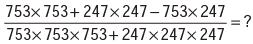Solution:

Given Expression =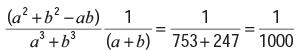QUESTION: 4

What is the value of given number expression? 107 x 107 + 93 × 93 = ?

Solution:

107 × 107 + 93 × 93 = (107)2 + (93)2
= (100 + 7)2 + (100 – 7)2
= 2 × [(100)2 + 72]       [Ref: (a + b)2 + (a – b)2
= 2(a2 + b2)] = 20098

QUESTION: 5

Which of the following is the remainder when we divide (6767 + 67) by 68?

Solution:

(xn + 1) will be divisible by (x + 1) only when n is odd.
(6767 + 1) will be divisible by (67 + 1)
(6767 + 1) + 66, when divided by 68 will give 66 as remainder.

QUESTION: 6

Find the sum of first 5 prime numbers.

Solution:

Required sum = (2 + 3 + 5 + 7 + 11) = 28.

QUESTION: 7

Replace question mark with the suitable answer:

(12345679 × 2 × 62) = ?

Solution:

(12345679 × 2 × 62)  = 12345679 × 72
= 12345679 × (70 +2)
= 12345679 × 70 + 12345679 × 2
= 864197530 + 24691358
= 888888888

QUESTION: 8

Find the value of n if (64)2 – (36)2 = 20 × n.

Solution:

20 × n = (64 + 36)(64 – 36) = 100 × 28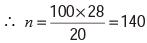QUESTION: 9

Which of the following is the correct value of given number expression: (22 + 42 + 62 + ... + 202) = ?

Solution:

(22 + 42 + 62 + ... + 202
= (1 × 2)2 + (2 × 2)2 + (2 × 3)2 + ... + (2 × 10)2
= (22 × 12) + (22 × 22) + (22 × 32) + ...
+ (22 × 102)
= 22 × [12 + 22 + 32 + ... + 102]
[Formula: (12 + 22 + 32 + ... + n2)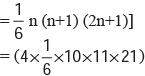= (4 × 5 × 77)
= 2 × 77 × 10

QUESTION: 10

What will be the sum of first forty five counting numbers?

Solution:

Let Sn = (1 + 2 + 3 + ... + 45)
This is an A.P. in which a = 1, d = 1, n = 45 and l = 45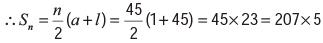Required sum = 207 × 5.

QUESTION: 11

What will be the sum of even numbers between 1 and 21 is?

Solution:

Let Sn = (2 + 4 + 6 + ... + 20).
This is an A.P.
in which a = 2, d = 2 and l = 20
Let the number of terms be n. Then,
a + (n – 1)d = 20
2 + (n – 1) × 2 = 20
n = 10.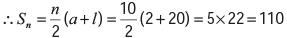QUESTION: 12

What is the value obtained if we multiply 2056 and 987?

Solution:

2056 × 987 = 2056 × (1000 – 13)
= 2056 × 1000 – 2056 × 13
= 2056000 – 26728
= 2029272

QUESTION: 13

If two-third of three-fourth of a number is 30, then three – fifth of that number is:

Solution:

Let the number be x.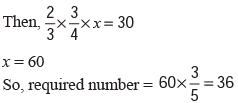QUESTION: 14

What is the value of third integer if 3 times the first of 3 odd consecutive integers is three more than twice the third?

Solution:

Let the three integers be x, x + 2 and x + 4.
Then, 3x = 2(x + 4) + 3
x = 11
Third integer = x + 4 = 15

QUESTION: 15

Find the value of given number expression: 397 × 397 + 104 × 104 + 2 × 397 × 104 = ?

Solution:

Given number expression = (397)2 + (104)2 + 2 × 397 × 104
= (397 + 104)2
= (501)2 = (500 + 1)2
= (500)2 + (1)2 + (2 × 500 × 1)
= 250000 + 1 + 1000
= 251001

QUESTION: 16

Which of the following value is true in place of question mark? (35423 + 7164 + 41720) – (317 × 89) = ?

Solution:

(35423 + 7164 + 41720) – (317 × 89)
= (35423 + 7164 + 41720) – (28213)
= 84307 – 28213
= 56094

QUESTION: 17

(an – bn) is completely divisible by (a – b), when

Solution:

For every natural number n, (an – bn) is completely divisible by (a – b).

QUESTION: 18

Find the divisor by which the given number expression (325 + 326 + 327 + 328) is completely divided.

Solution:

(325 + 326 + 327 + 328) = 325 × (1 + 3 + 32 + 33)
= 325 × 40
= 324 × 3 × 4 × 10
= (324 × 4 × 30), which is divisible by 30.

QUESTION: 19

When we divide a number say x by 6 it leaves a remainder 3. When we divide the square of the same number by 6, what will be the remainder?

Solution:

Let x = 6q + 3.
Then, x2 = (6q + 3)2
= 36q2 + 36q + 9
= 6(6q2 + 6q + 1) + 3
Thus, when x2 is divided by 6, then remainder = 3.

QUESTION: 20

Which of the following is the remainder when we divide 17200 by 18?

Solution:

When n is even; (xn- an) is completely divisible by (x + a)
(17200 – 1200) is completely divisible by (17 + 1), i.e., 18.
(17200 – 1) is completely divisible by 18.
On dividing 17200 by 18, we get 1 as remainder.